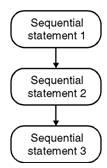Home > VHDL > Sequential Statements

Introduction to Sequential Statements :

You can use sequential statements only inside a process statement or within a subprogram (procedure or function). Each statement executes in the order in which it is encountered. The preceding BNF description listed the sequential statements available in VHDL. Sequential statements are divided into categories, based on their operation. The figure below shows the categories for the sequential statements.The types of sequential statements are

* Assignment Statements and Targets

• Variable Assignment Statements

* Signal Assignment Statements

• If Statements
• Case Statements
• Loop Statements
• Next Statements
• Exit Statements
• Subprograms
• Return Statement
• Wait Statements

Null Statements

Assignment Statements and Targets :

Use an assignment statement to assign a value to a variable or signal. The syntax is :

target := expression;

target <= expression;

The difference in syntax between variable assignments and signal assignments is that

â€“ Variables use the: = operator

Variables are local to a process or subprogram, and their assignments take effect immediately.

â€“ Signals use the <= operator

Signals need to be global in a process or subprogram, and their assignments take effect at the end of a process. Signals are the only means of communication between processes.

Variable Assignment Statements :

A variable assignment changes the value of a variable. The syntax is :

target : = expression;

Signal Assignment Statements :

A signal assignment changes the value being driven on a signal by the current process. The syntax is :

target <= expression;

Ã˜ Example : Variable and Signal Assignments

signal K1, K2 : STD_LOGOC;

signal K_OUT : STD_LOGIC_VECTOR(1 to 8);

. . .

process ( K1, K2 )

variable V1, V2: STD_LOGIC;

begin

V1 := '1'; -- This sets the value of V1

V2 := '1'; -- This sets the value of V2

K1 <= '1'; -- This assignment is the driver for K1

K2 <= '1'; -- This has no effect because of the

-- Assignment later in this process

K_OUT(1) <= V1; -- Assigns '1', the value assigned above

K_OUT(2) <= V2; -- Assigns '1', the value assigned above

K_OUT(3) <= K1; -- Assigns '1', the value assigned above

K_OUT(4) <= K2; -- Assigns '0', the value assigned below

V1 := '0'; -- This sets the new value of V1

V2 := '0'; -- This sets the new value of V2

K1 <= '0';

K2 <= '0'; -- This assignment overrides the previous

-- One since it is the last assignment to

-- This signal in this process

K_OUT(5) <= V1; -- Assigns '0', the value assigned above

K_OUT(6) <= V2; -- Assigns '0', the value assigned above

K_OUT(7) <= K1; -- Assigns '1', the value assigned above

K_OUT(8) <= K2; -- Assigns '0', the value assigned above

end process ;